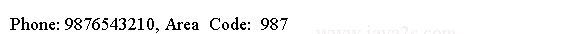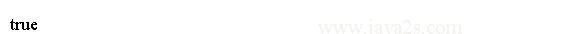# Java Regular Expression Tutorial - Java Regex Quantifiers

We can specify the number of times a character in a regular expression may match the sequence of characters.

To express a pattern "one digit or more" using a regular expression, we can use the quantifiers.

Quantifiers and their meanings are listed in the following Table.

QuantifiersMeaning
*Zero or more times
+One or more times
?Once or not at all
{m}Exactly m times
{m,}At least m times
{m, n}At least m, but not more than n times

The quantifiers must follow a character or character class.

## Example

```import java.util.regex.Matcher;
import java.util.regex.Pattern;
//from w  ww  . java 2s  .co m
public class Main {
public static void main(String[] args) {
// A group of 3 digits followed by 7 digits.
String regex = "\\b(\\d{3})\\d{7}\\b";

// Compile the regular expression
Pattern p = Pattern.compile(regex);

String source = "12345678, 12345, and 9876543210";

// Get the Matcher object
Matcher m = p.matcher(source);

// Start matching and display the found area codes
while (m.find()) {
String phone = m.group();
String areaCode = m.group(1);
System.out.println("Phone: " + phone + ", Area  Code:  " + areaCode);
}
}
}
```

The code above generates the following result.## Example 2

`*` matches zero or more `d`.

```import java.util.regex.Pattern;
//  w  ww .  j  a v a  2 s . c  om
public class Main {
public static void main(String args[]) {